# A Novel Cascade Binary Tagging Framework for Relational Triple Extraction

## Basic Idea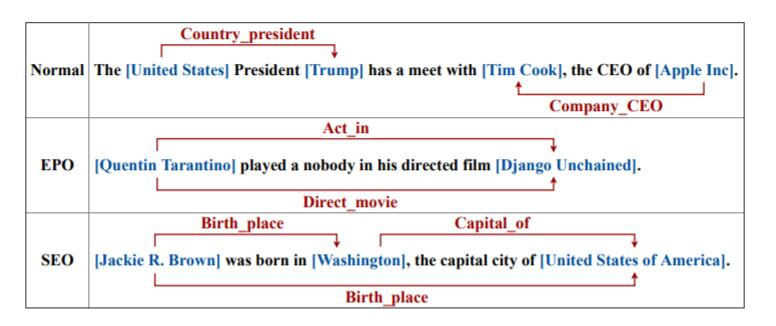• EPO(Entity Pair Overlap): 实体对重叠是指实体对之间具有多种关系, 这些三元组共享相同的实体.
• SEO(Single Entity Overlap): 单实体重叠是指有单个实体被多个三元组共享, 如例子中的Washington.

## CasRel

CasRel与先前的模型不同, 它希望将目标函数指定在Triple Level, 而非像先前的做法将三元组抽取的过程拆开, 并分别设立目标函数.

\begin{aligned} & \prod_{j=1}^{|D|}\left[\prod_{(s, r, o) \in T_{j}} p\left((s, r, o) \mid x_{j}\right)\right] \\ =& \prod_{j=1}^{|D|}\left[\prod_{s \in T_{j}} p\left(s \mid x_{j}\right) \prod_{(r, o) \in T_{j} \mid s} p\left((r, o) \mid s, x_{j}\right)\right] \\ =& \prod_{j=1}^{|D|}\left[\prod_{s \in T_{j}} p\left(s \mid x_{j}\right) \prod_{r \in T_{j} \mid s} p_{r}\left(o \mid s, x_{j}\right) \prod_{r \in R \backslash T_{j} \mid s} p_{r}\left(o_{\varnothing} \mid s, x_{j}\right)\right] \end{aligned}

1. Triple Level的目标函数建立与最终评估方式是相符的.
2. Triple Level的建模直接规避了实体如何被共享的问题.
3. 第三步的拆解启发了模型的结构设计. 通过最大化$p\left(s \mid x_{j}\right)$训练Subject Tagger, 再在已知Subject的条件下最大化给定关系$r$ 的$p_{r}\left(o \mid s, x_{j}\right)$ 来训练Object Tagger, 关系便自然成为实体之间的映射函数.

### BERT Encoder

\begin{aligned} \mathbf{h}_{0} &=\mathbf{S} \mathbf{W}_{s}+\mathbf{W}_{p} \\ \mathbf{h}_{\alpha} &=\operatorname{Trans}\left(\mathbf{h}_{\alpha-1}\right), \alpha \in[1, N] \end{aligned}

$\mathbf{S}$ 为句子的One Hot向量, $\mathbf{W}_s$ 为Subword的Embedding矩阵, $\mathbf{W}_p$ 为位置编码矩阵.

1. 首先从句子中抽取Subject.
2. 然后在所有可能的关系$r$ 的条件下, 看是否有与Subject相对应的Object.

#### Subject Tagger

$$\begin{array}{r} p_{i}^{\text {start_s }}=\sigma\left(\mathbf{W}_{\text {start }} \mathbf{x}_{i}+\mathbf{b}_{\text {start }}\right) \\ p_{i}^{\text {end_s }}=\sigma\left(\mathbf{W}_{\text {end }} \mathbf{x}_{i}+\mathbf{b}_{\text {end }}\right) \end{array}$$

$p_{i}^{\text {start_s }}, p_{i}^{\text {end_s }}$ 分别为输入句子的第$i$ 个Token是否为Subject起始位置的概率和结束位置的概率.

$$p_{\theta}(s \mid \mathbf{x}) =\prod_{t \in\{\text { start_s }, \text { end_s }\}} \prod_{i=1}^{L}\left(p_{i}^{t}\right)^{\mathbf{I}\left\{y_{i}^{t}=1\right\}}\left(1-p_{i}^{t}\right)^{\mathbf{I}\left\{y_{i}^{t}=0\right\}}$$

$L$ 为句长, $\mathbf{I}\set{z} = 1$ 表示如果$z$ 为真则为1, 否则为0. $y_i^{\text {start_s }}, y_i^{\text {end_s }}$ 分别为Subject的起始结束位置二值化的Tag. $\theta$ 就是前面提到的Subject Tagger的参数.

Subject Tagger如下图所示: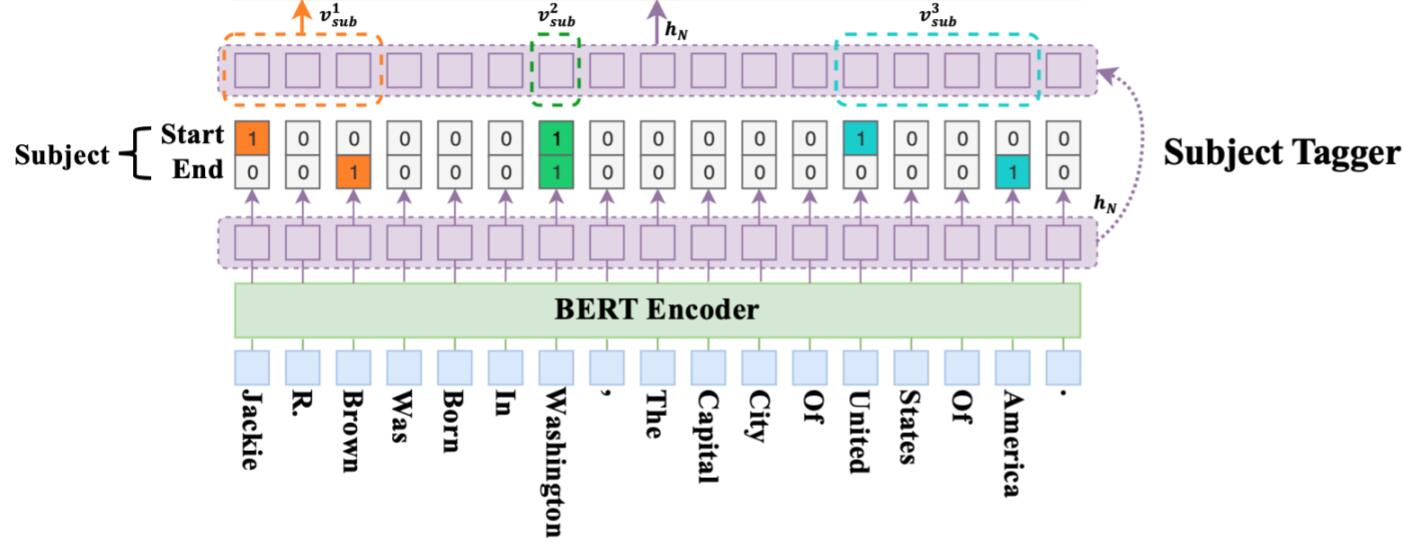#### Relation - specific Object Taggers

$$\begin{array}{r} p_{i}^{\text {start_o }}=\sigma\left(\mathbf{W}_{\text {start }}^{r}\left(\mathbf{x}_{i}+\mathbf{v}_{\text {sub }}^{k}\right)+\mathbf{b}_{\text {start }}^{r}\right) \\ p_{i}^{\text {end_o }}=\sigma\left(\mathbf{W}_{\text {end }}^{r}\left(\mathbf{x}_{i}+\mathbf{v}_{s u b}^{k}\right)+\mathbf{b}_{\text {end }}^{r}\right) \end{array}$$

$p_{i}^{\text {start_o }}, p_{i}^{\text {end_o }}$ 分别为输入句子的第$i$ 个Token是否为Object起始位置的概率和结束位置的概率. 这里作者简单的取了第$k$ 个Subject所有Token的平均$\mathbf{v}^k_{sub}$ 作为Subject传递的信息.

$$p_{\phi_{r}}(o \mid s, \mathbf{x}) =\prod_{t \in\{\text { start_o, end_o }\}} \prod_{i=1}^{L}\left(p_{i}^{t}\right)^{\mathbf{I}\left\{y_{i}^{t}=1\right\}}\left(1-p_{i}^{t}\right) ^\mathbf{\mathbf { I } \{ y _ { i } ^ { t } = 0 \}}$$

$y_i^{\text {start_o }}, y_i^{\text {end_o }}$ 分别为Object的起始结束位置二值化的Tag. $\phi_r$ 为关系特化的Object Tagger的参数.

Relation - specific Object Taggers如下图所示: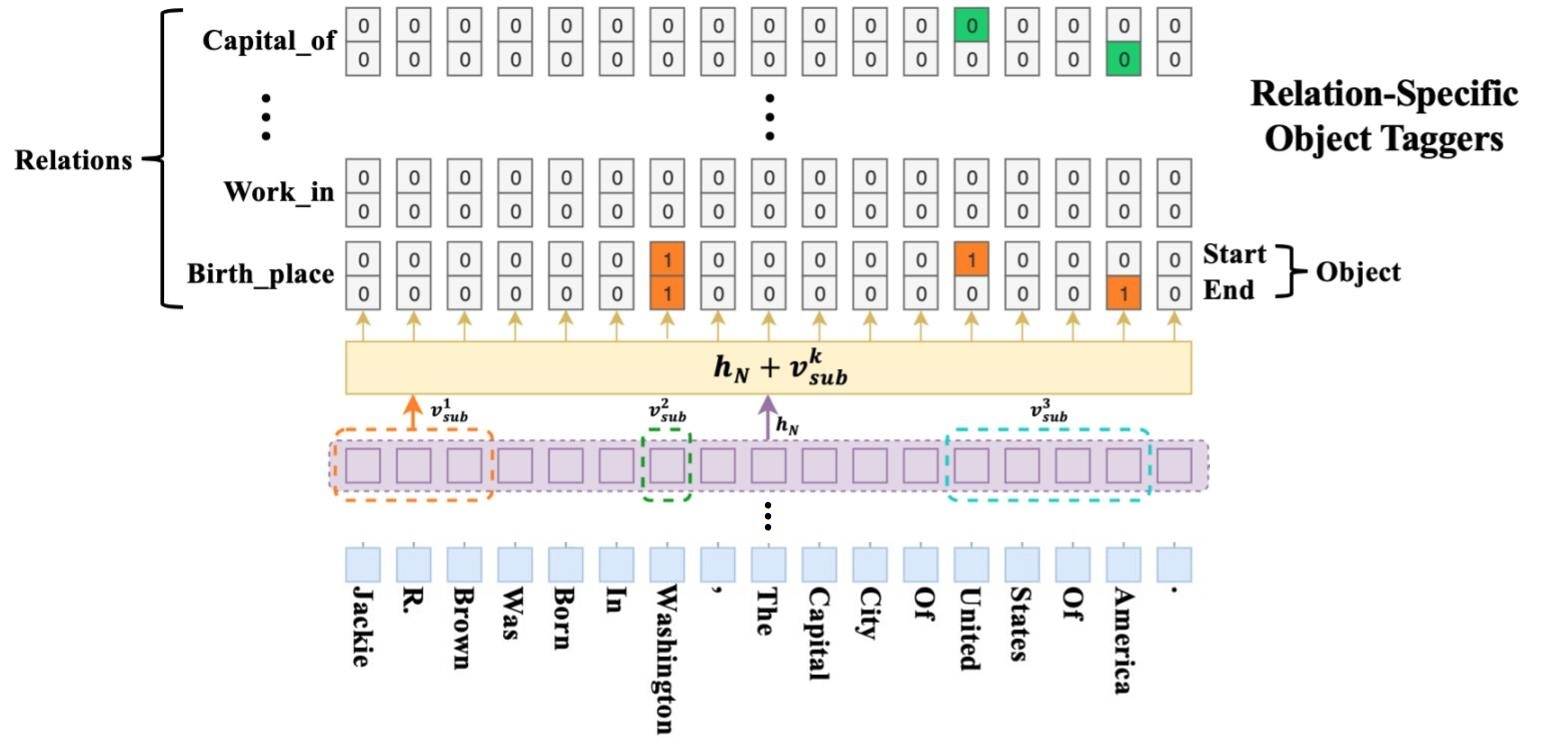CasRel会在不同的Subject之间迭代. 从Subject Tagger获取了指定的实体后, 简单的将第$k$ 个实体所在区域的Token取平均得到$\mathbf{v}^k_{sub}$, 与BERT抽取出的$\mathbf{h}_N$ 相加, 以此判断句子中所有位置是否有可能含有给定关系$r$ 的Object, 并用起始位置和结束位置来标识Object.

### Data Log-likelihood Objective

$$J(\Theta) = \sum_{j=1}^{|D|}\left[\sum_{s \in T_{j}} \log p_{\theta}\left(s \mid \mathbf{x}_{j}\right)+\sum_{r \in T_{j} \mid s} \log p_{\phi_{r}}\left(o \mid s, \mathbf{x}_{j}\right)+\sum_{r \in R \backslash T_{j} \mid s} \log p_{\phi_{r}}\left(o_{\varnothing} \mid s, \mathbf{x}_{j}\right)\right]$$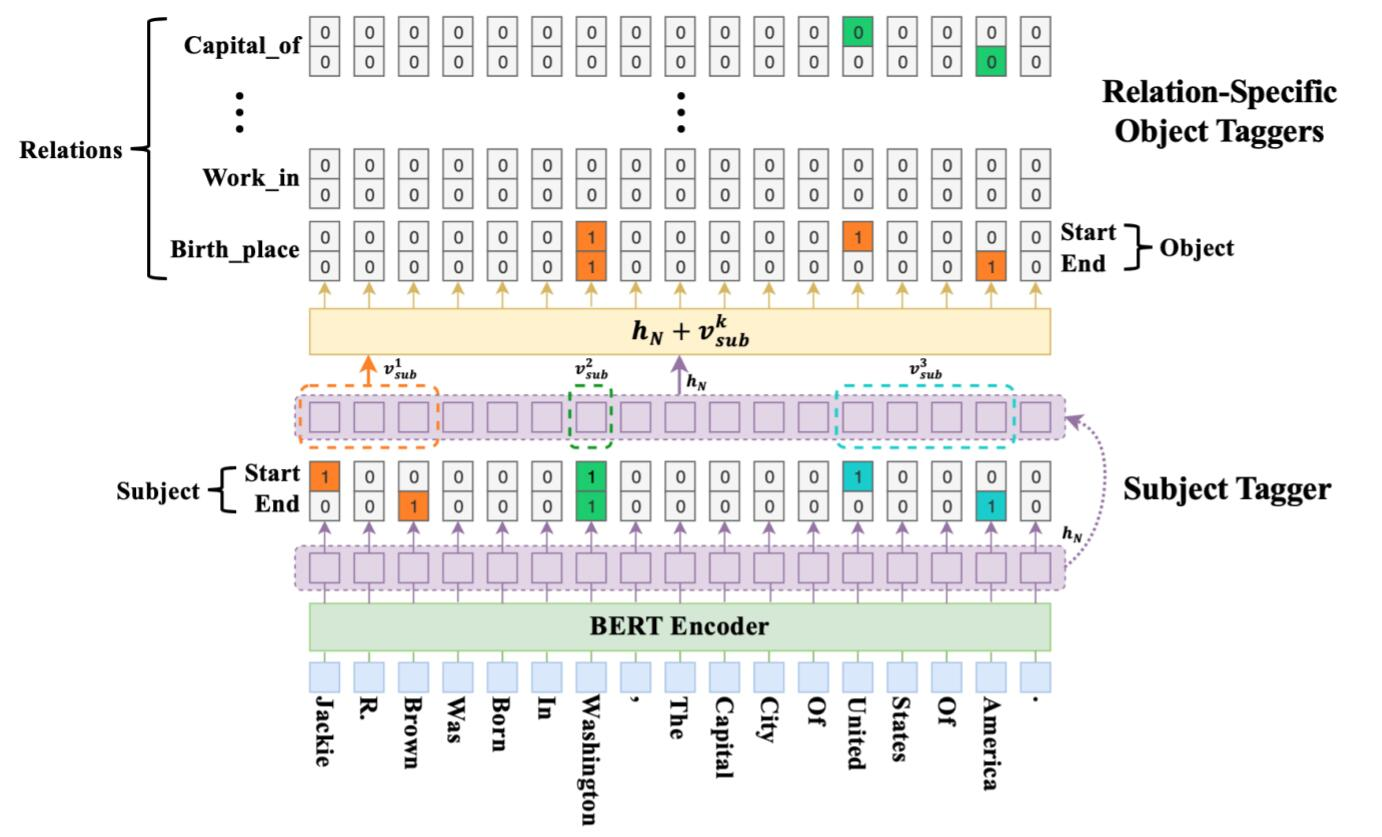## Experiments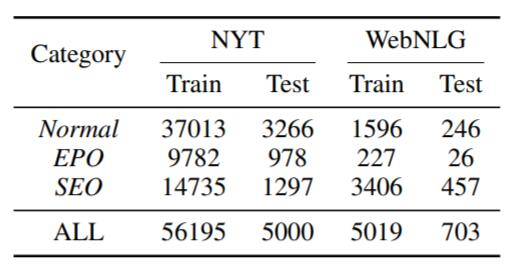### Main Results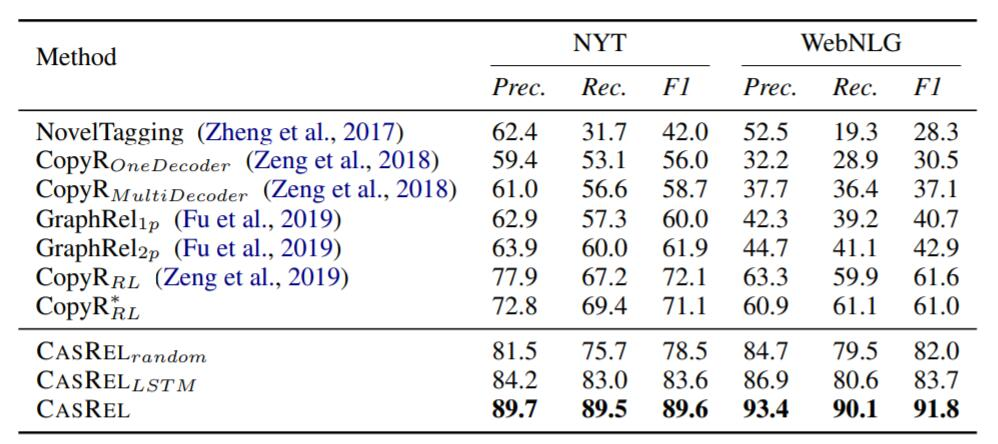### Detail Results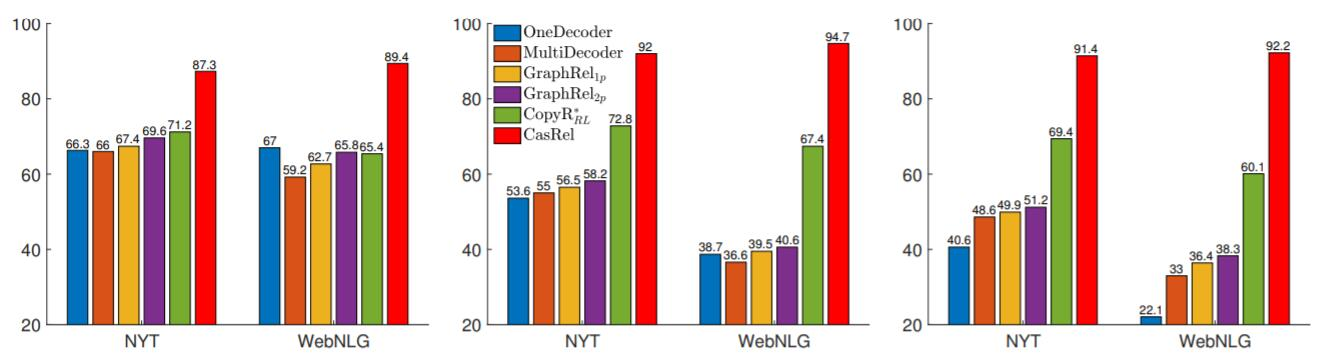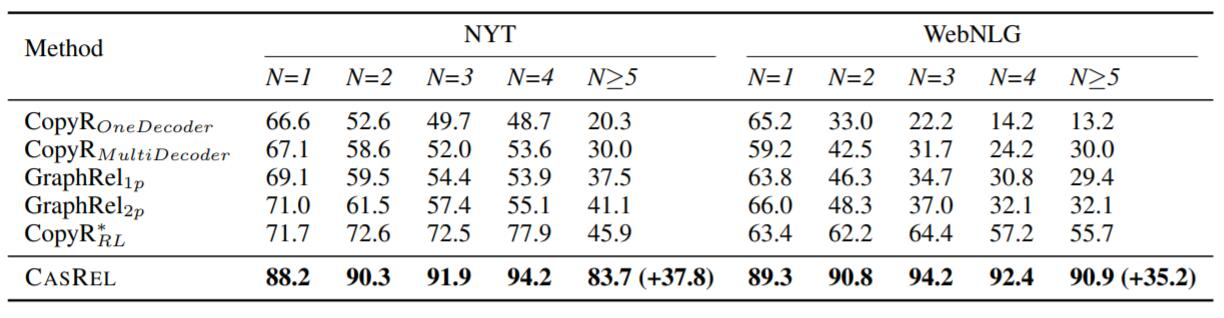## Summary

上一篇A Unified MRC Framework for Named Entity Recognition

2021-09-30
目录